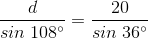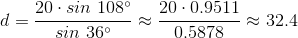# Intermediate Geometry : How to find the length of the diagonal of a pentagon

## Example Questions

### Example Question #1 : How To Find The Length Of The Diagonal Of A Pentagon

In the pentagon below, the length of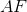is one-third that of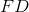. Find the length of diagonal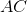.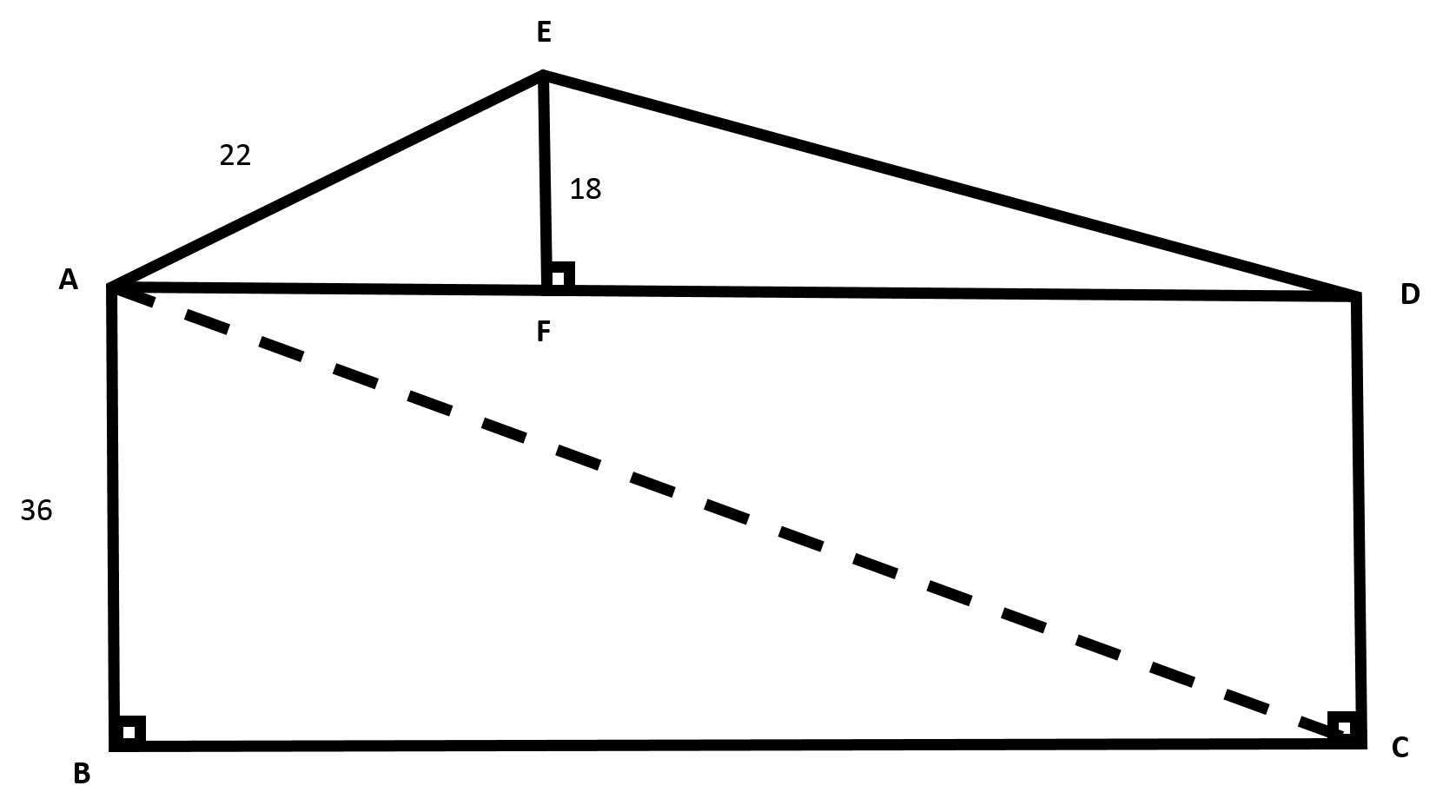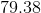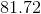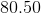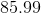Explanation:Start by using the Pythagorean theorem to find the length of.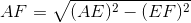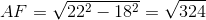Sinceis one-third the length of, we know that the length ofmust be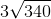.

Now, notice that the pentagon is made up of one rectangle and two right triangles.

Focus on rectangle. We can find the length of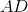by adding together the lengths ofand.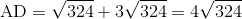Since we are working with a rectangle, we know that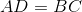. Thus, we can use the Pythagorean Theorem again to find the length of.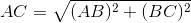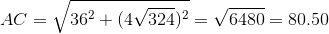Make sure to round to two places after the decimal.

### Example Question #2 : How To Find The Length Of The Diagonal Of A Pentagon

What is the length of a diagonal of a pentagon with side length of 20 centimeters (to the nearest tenth)?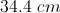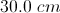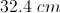Explanation:

Letbe the length fo a diagonal.

One interior angle of a pentagon measures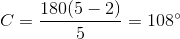A diagonal of this pentagon, along with two sides, forms an isosceles triangle with sides 20, 20 and; the side of lengthis opposite the angle of measure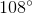, and each side of length 20 is opposite an angle of measure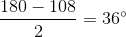.

The Law of Sines helps us to determine: